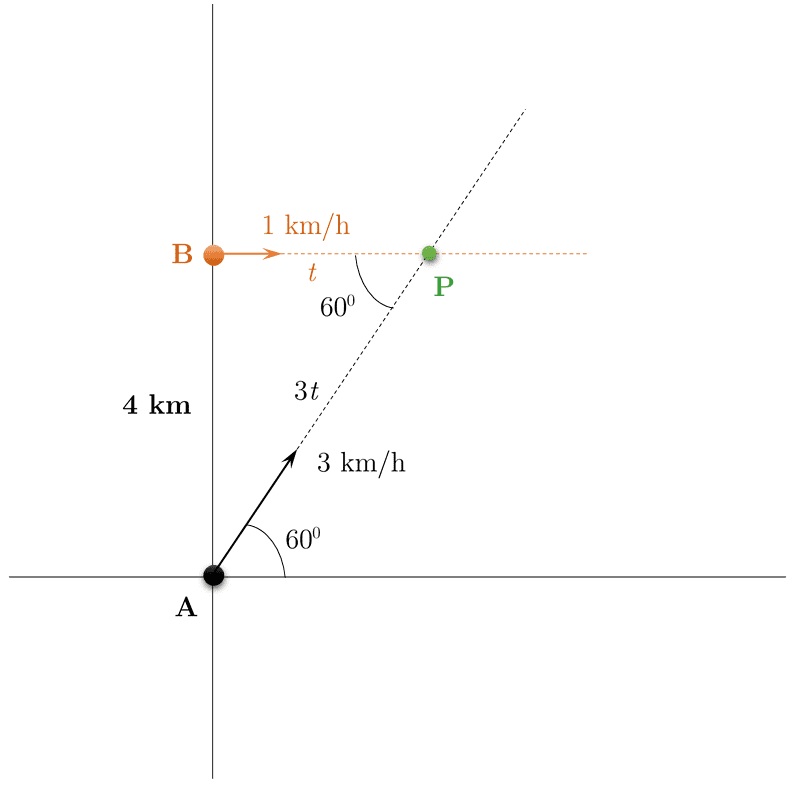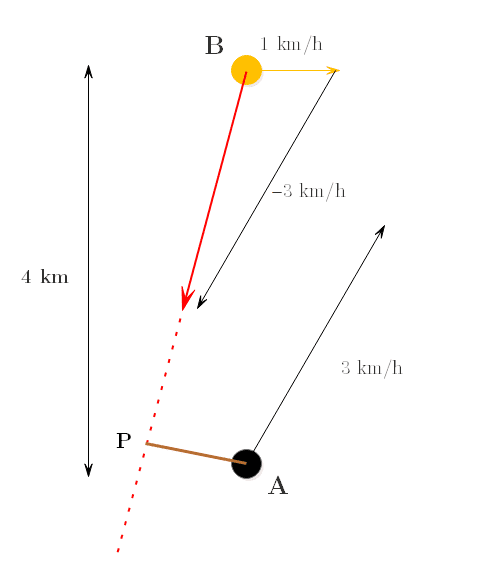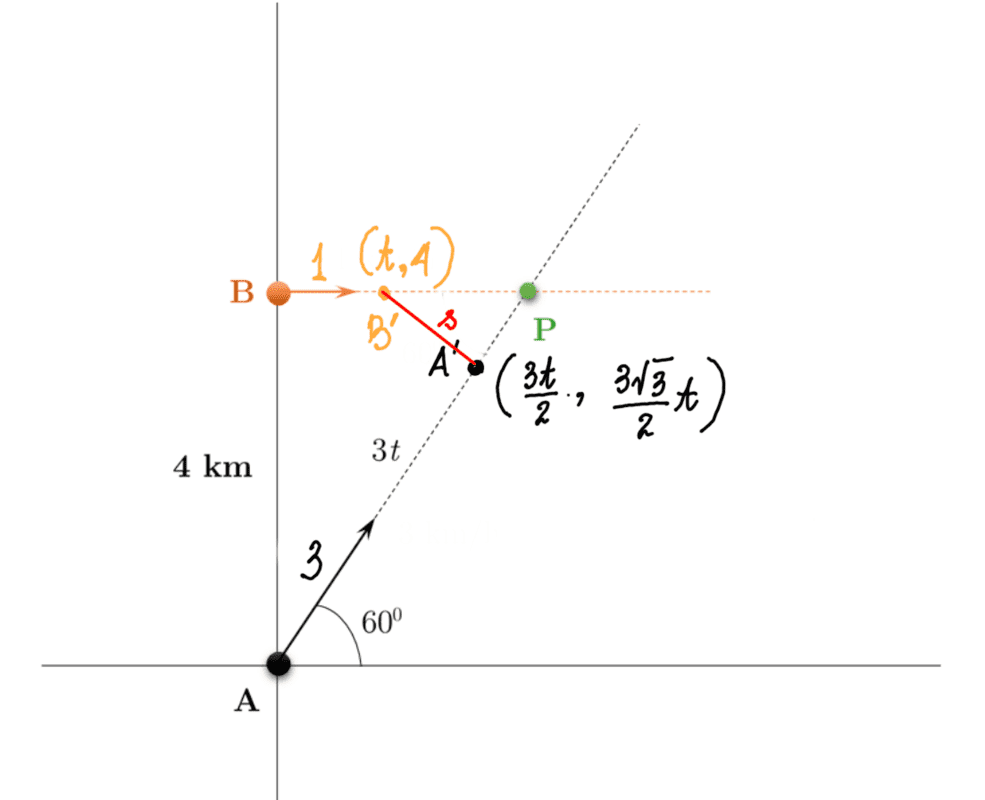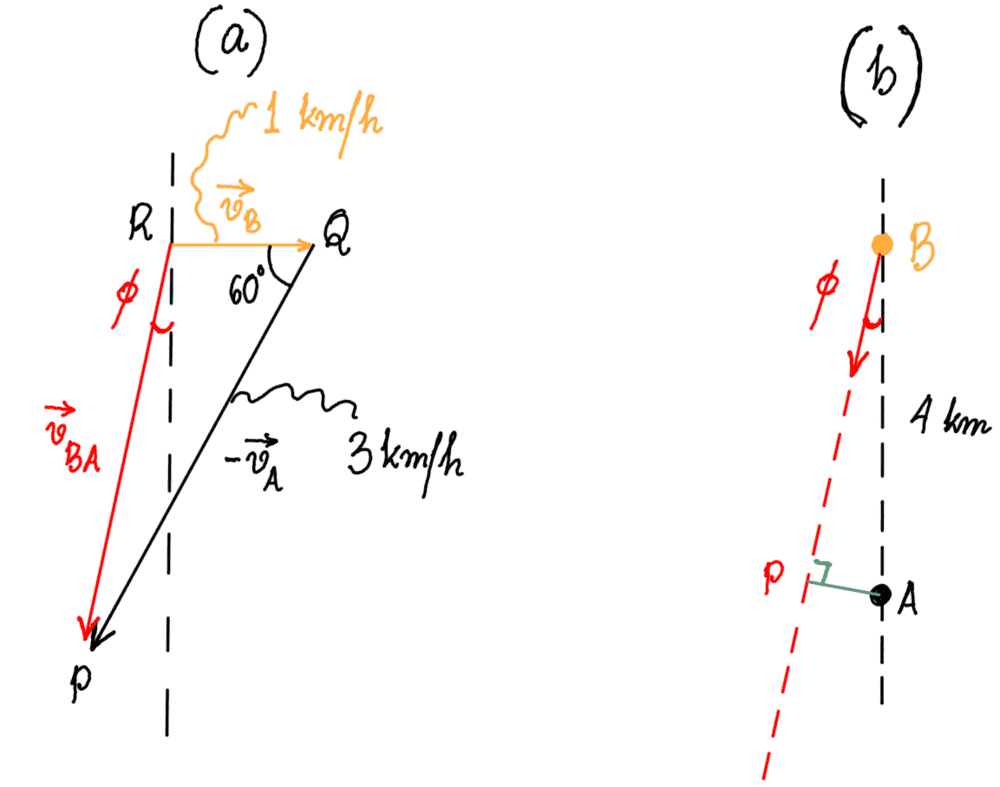# Two ships - will they collide?

Homework Statement:
Two ships A and B begin at the same time from their respective points 4 kms apart along the ##y## axis with speeds 3 km/h and 1 km/h (uniformly) respectively, as shown in the figure below. The motion of ship B is parallel to the ##x## axis while that of A is at an angle of ##60^{\circ}## to the ##x## axis. ##\textbf{Will the two ships collide at point P}?##
Relevant Equations:
For unaccelerated motion, distance travelled ##d = v_0 t##, where ##v_0## is the uniform speed. Also, in a right angled triangle, ##\cos \theta = \frac{\text{adjacent}}{\text{hypotenuse}}##.There are two ways to solve. My problem is, when I apply the two, I end up with a contradiction.

Method 1 : Conclusion - Ships will not collide .

Imagine the ships collide after a time ##t##.

Since they both start at the same time, the distances travelled by them would be ##3t## (A) and ##t## (B), respectively. (I have shown the two distances in the diagram, in terms of ##t##).

Using parallel-line and angles, we see that ##\angle BPA = 60^{\circ}##. Thus if we take the cosine of the angle using trigonemetrical ratios of a right angled triangle, we have ##\cos 60^{\circ} = \frac{t}{3t} = \frac{1}{3}##!

We know that ##\cos 60^{\circ} = \frac{1}{2}##, hence it's clear that two two ships will not collide .

Method 2 : Conclusion - Ships will collide.

Imagine the ships collide after a time ##t##.

Since they both start at the same time, the distances travelled by them would be ##3t## (A) and ##t## (B), respectively. (I have shown the two distances in the diagram, in terms of ##t##).

In the right angled ##\triangle ABP##, using the Pythagorean theorem, we have ##(3t)^2 = t^2 + 4^2 \Rightarrow 9t^2 = t^2 + 16 \Rightarrow 8t^2 = 16 \Rightarrow t^2 = 2 \Rightarrow t = \sqrt{2}\; \text{hr} \approx 85 \text{minutes}## .

Hence the two ships will collide after a time of 85 minutes from start.

One possible way out is to note that nowhere in my second solution did I consider the fact that Ship A was moving at an angle of 60 degrees to the horizontal. What I did show was that with the speeds as given, the two ships can collide after the given time (85 minutes). If that was to happen, then the angle at which Ship A should move would be ##\cos^{-1} \frac{t}{3t} = \cos^{-1} \frac{1}{3} = 70.5^{\circ}##. It becomes a different problem really : Given the speeds of 1 and 3 km/h, calculate the angle at which ship A should move in order that they collide.

Am I right? When I drew the relative velocity of B with respect to A (##\vec v_{BA}##), I found that it was pointing away from A, clearly showing that ship B would not be moving in A's direction and that no collision would take place.

BvU
Homework Helper
Am I right?
Yes
I found that it was pointing away from A
You mean: it was not pointing directly at A:
if it were pointing away from A, the ships would not come closer than the original 4 km, but they do!

You mean: it was not pointing directly at A:
if it were pointing away from A, the ships would not come closer than the original 4 km, but they do!

Yes, I paste a small diagram of how the relative velocity of B with respect to A (##\vec {v}_{BA}##) looks like :As you can see above, ##\vec {v}_{BA}## is given by the red arrow. Yes, of course I meant the relative velocity of B w.r.t. A is not pointing directly at A. I suppose that is enough to imply that the ships won't collide.

AP is the distance of shortest approach. I suppose it will be instructive of me to calculate how much AP is?

BvU
Homework Helper
I suppose it will be instructive of me to calculate how much AP is?
Yes: in practice (boats, planes) a safety margin is to be observed. Do you know how to find this distance ? I get 0.76 km

gmax137
Method 1 : Conclusion - Ships will not collide .

Imagine the ships collide after a time ##t##.

Method 2 : Conclusion - Ships will collide.

Imagine the ships collide after a time ##t##.

I think it would be much simpler to
1. determine the coordinates (x,y) of point P (this is simple trig). This allows you to figure the distance AP and the distance BP.
2. determine how long Ship A takes to reach P
3. determine how long Ship B takes to reach P

If the times are the same, then they arrive together (ie, collide). If the times are different, one ship gets there first (ie, they do not collide).

kuruman
Homework Helper
Gold Member
Just a minor point that might prevent confusion and frustration in the future. In the drawing in post #3 all arrows representing vectors should be labeled with their magnitudes which, in this case, are speeds. There should be no -3 km/h label. The convention is that a vector drawn graphically as an arrow needs a label to convey the length of its shaft (magnitude) and an arrowhead to convey its direction. Negative signs come in when you transit from the graphic to the algebraic representation. In this case you would write,
##\vec v_1=3~\mathrm{km/h}~\cos(60^o)~\hat i+3~\mathrm{km/h}~\sin(60^o)~\hat j##
and
##\vec v_2=3~\mathrm{km/h}~\cos(240^o)~\hat i+3~\mathrm{km/h}~\sin(240^o)~\hat j##

It is clear that, when you do it formally and measure angles counterclockwise from the positive ##x##-axis, the negative sign is associated with the component(s) and not the magnitude of a vector. The arrowheads are there to help you figure out the correct angle. Had you used vector symbols instead of scalar numbers it would have been OK to label one of the arrows with ##\vec v_1## and the other with ##-\vec v_1##. Then it's clear that you are labeling the whole arrow and not just its shaft.

Last edited:
•BvU
Yes: in practice (boats, planes) a safety margin is to be observed. Do you know how to find this distance ? I get 0.76 km

Yes. I have found two ways to find the distance of closest approach and the answer matches yours. Nonetheless, please see my working below to let me know if they are ok.

[Find the distance of closest approach for the two ships in the problem above and the time at which they are at their closest]

First Method : (Mathematical)(Please note that all time given above are in hours and all distances in kilometers. Speeds are in km/h).

After a time period of ##t##, the coordinates of ship B are ##(t,4)## at the point B' and those of ship A are ##(\frac{3}{2}t, \frac{3\sqrt{3}}{2}t)## where the distance travelled by A is ##AA' = 3t## and elementary trigonometry is used to find its coordinates at A'.

The distance between the ships ##A'B' = s(t)## where ##s^2 = \frac{t^2}{4}+\left(\frac{3\sqrt{3}}{2}t - 4\right)^2##.

Differentiating s with respect to t and putting ##\frac{ds}{dt} = 0## for conditions of extrema, we are left with,
##0 = \frac{t}{2}+\frac{27}{2}t-12\sqrt{3} \Rightarrow 14t = 12\sqrt{3} \Rightarrow t = \frac{6}{7}\sqrt{3}\Rightarrow \boxed{t = 1.48\; \text{hr}}##.

The closest distance ##s_{\text{min}} = \left(\frac{1.48^2}{4} + \left(\frac{3\sqrt{3}}{2}\times 1.48-4\right)^2 \right)^{\frac{1}{2}}\Rightarrow \boxed{s_{\text{min}}= 0.76\; \text{km}}##.

(The necessity to prove that the distance so found is a minima is perhaps superfluous. Clearly the distance cannot be a maxima because the two ships can continue moving further and the maximum distance of their separation is ##\infty##. However, if the reader does want to really show that the distance is a minima, the above expression for ##s## needs to be differentiated again to find ##\frac{d^2s}{dt^2} = \frac{7}{s_{\text{min}}} >0## (minima)

Second Method : (Physical)First let's find the velocity of B relative to A ##\vec v_{BA}##. In the right angled triangle PQR in figure (a) above, we know from elementary trigonometry that ##v_{BA} = (v_A^2 + v_B^2 - 2 v_A v_B \cos \theta)^{\frac{1}{2}} = (9+1-2\times 3\times 1\times \frac{1}{2})^{\frac{1}{2}} = 7^{\frac{1}{2}} = 2.645\; \text{km/h}##.
Using the "sine rule" to triangle PQR and noting the angle ##\phi##, we have ##\frac{3}{\sin(90+\phi)} = \frac{2.465}{\sin 60}\Rightarrow \phi = 10.81^{\circ}##.

Moving over to diagram on side(b), the distance of closest approach must be the perpendicular from A = AP. Using trigonometry, ##AP = 4 \sin\phi = 4\times \sin 10.81 \Rightarrow \boxed{AP = 0.75\; \text{km}}##.

Using trigonometry again, the distance travelled by B in A's frame (Very important = This is not the same as the distance travelled by B in the earth's frame at closest approach. Please note: the distance between non-simultaneous events is not an invariant between frames in uniform motion) : ##BP = 4 \cos 10.81 = 3.93\; \text{km}##.

Hence the time for closest approach : ##t = \frac{BP}{v_{BA}} = \frac{3.93}{2.645} \Rightarrow \boxed{t = 1.48\; \text{hr}}##.